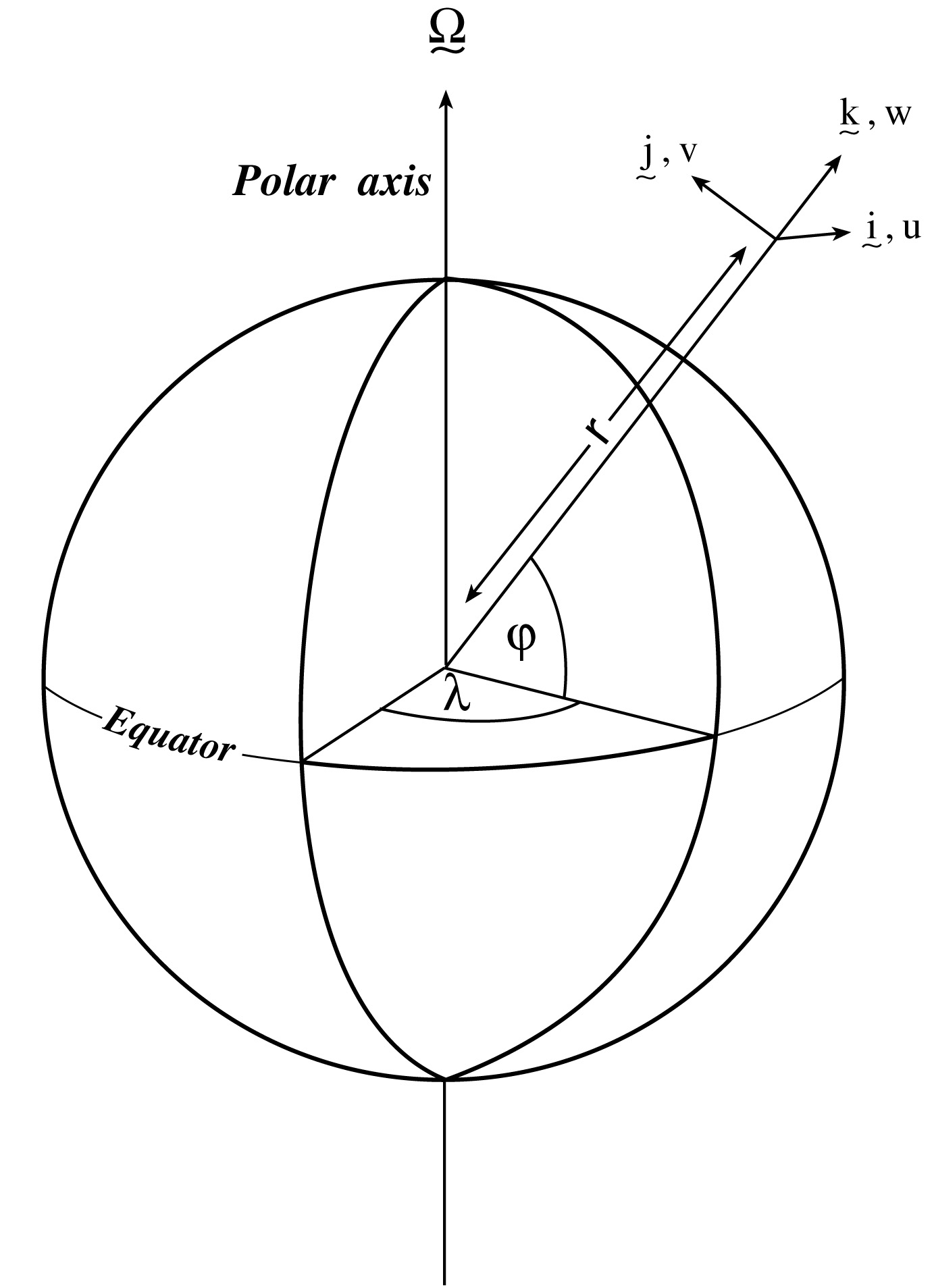# 1.6.1. Coordinate systems¶

## 1.6.1.1. Spherical coordinates¶

In spherical coordinates, the velocity components in the zonal, meridional and vertical direction respectively, are given by:

$u=r\cos \varphi \frac{D\lambda }{Dt}$
$v=r\frac{D\varphi }{Dt}$
$\dot{r}=\frac{Dr}{Dt}$

(see Figure 1.20) Here $$\varphi$$ is the latitude, $$\lambda$$ the longitude, $$r$$ the radial distance of the particle from the center of the earth, $$\Omega$$ is the angular speed of rotation of the Earth and $$D/Dt$$ is the total derivative.

The ‘grad’ ($$\nabla$$) and ‘div’ ($$\nabla \cdot$$) operators are defined by, in spherical coordinates:

$\nabla \equiv \left( \frac{1}{r\cos \varphi }\frac{\partial }{\partial \lambda } ,\frac{1}{r}\frac{\partial }{\partial \varphi },\frac{\partial }{\partial r} \right)$
$\nabla \cdot v\equiv \frac{1}{r\cos \varphi }\left\{ \frac{\partial u}{\partial \lambda }+\frac{\partial }{\partial \varphi }\left( v\cos \varphi \right) \right\} +\frac{1}{r^{2}}\frac{\partial \left( r^{2}\dot{r}\right) }{\partial r}$Figure 1.20 Spherical polar coordinates: longitude $$\lambda$$, latitude $$\varphi$$ and $$r$$ the distance from the center.¶